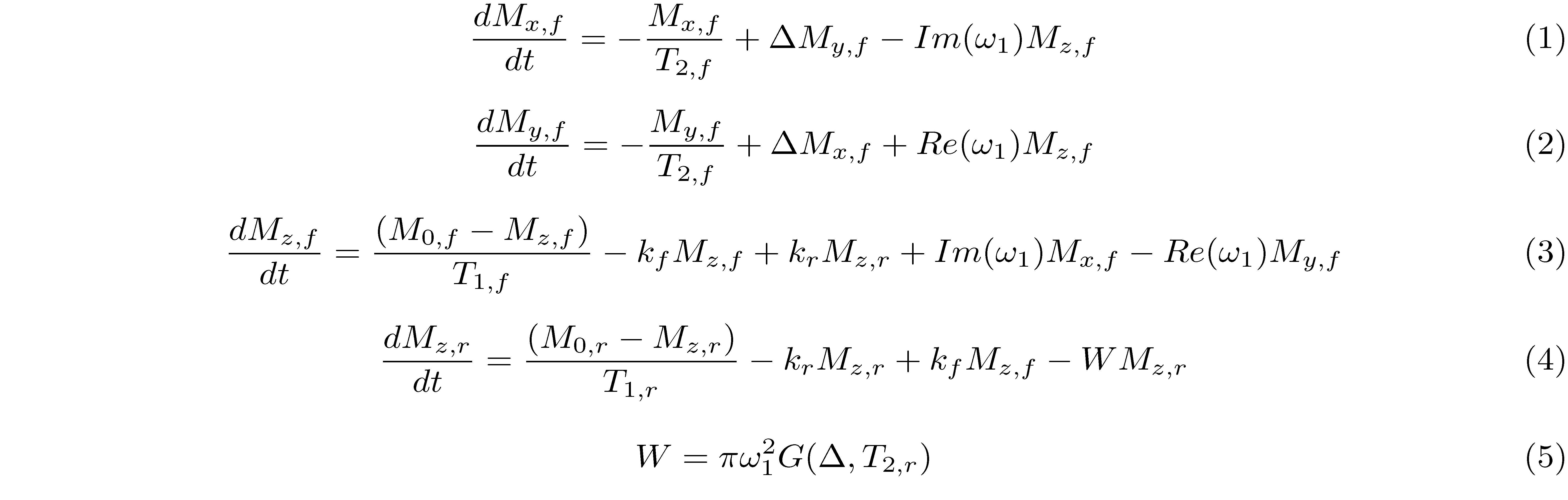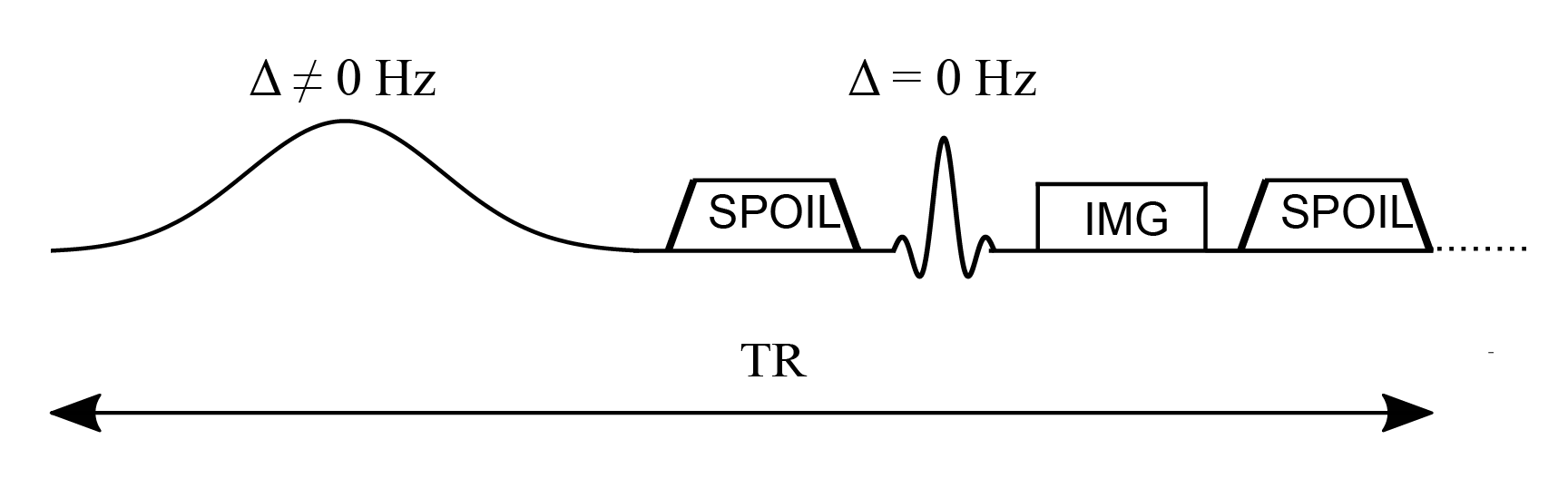# Quantitative Magnetization Transfer using Spoiled Gradient echo

Magnetization Transfer (MT) has benn extensively applied to study macromolecular biological tissue composition. The imaging contrast resides in the magnetization transfer between free-water protons and macromolecular proton compartments, through chemical exchange and dipolar interactions. In the two-pool tissue model, highly mobile protons are associated with the free-water pool while protons found in semisolid macromolecular sites are defined as the restricted pool (Sled 2018). A simple method for visualizing MT effects includes acquiring two images with and without an off-resonance MT pulse to calculate the MT ratio (MTR), which is the normalized difference of these two images (Wolff and Balaban 1989). Despite its proven usefulness to study multiple sclerosis (Zheng et al. 2018), Alzheimer’s disease (Fornari et al. 2012) and psychiatric disorders (Chen et al. 2015), the MTR is a semi-quantitative metric that depends critically on the imaging sequence parameters (Wood and Malik 2020). Another semi-quantitative approach is the estimation of MT saturation (MTsat) by fitting the MT signal obtained from an MT-weighted (MTw), proton density (PD) weighted and T1-weighted (T1w) contrast (Helms et al. 2008). Quantitative MT (qMT) consists of fitting multiple images to a mathematical model in order to extract tissue-specific parameters related to physical quantities, such as pool sizes, magnetization exchange rates between pools, and T1,T2 relaxation times of each pool. Compared to semi-quantitative approaches (MTR, MTsat), qMT has long acquisition protocols and sometimes needs additional measurements (eg. B0, B1, T1), and the complex models required to fit the quantitative maps makes it a challenging imaging technique.

In 2015, our lab published qMTLab (Cabana et al. 2015), an open-source software project seeking to unify three qMT methods in the same interface: qMT using spoiled gradient echo (qMT-SPGR), qMT using balanced steady-state free precession (qMT-bSSFP), and qMT using selective inversion recovery with fast spin echo (qMT-SIRFSE). qMTLab allowed users to simulate, evaluate, fit, and visualize qMT data with the possibility to share qMT protocols between researchers, allowing them to compare the performance of their methods (Cabana et al. 2015). Since then, we have extended the project and renamed it to qMRLab, (Karakuzu et al. 2020), which in addition to qMT now provides over 20 quantitative techniques under one umbrella, such as relaxation and diffusion models, quantitative susceptibility mapping, B0 and B1 mapping, etc. In addition, we published interactive tutorials (Boudreau 2018a; Boudreau 2018b; Boudreau 2019) and blog posts for several qMRI techniques that were published under creative commons licenses and some which have even been published in a book (Seiberlich et al. 2020). This blog post is a continuation of this outreach initiative, where we will focus on qMT and the tools we provide in qMRLab for this class of techniques.

Below is an introduction to qMT (with a focus on qMT-SPGR), where we will cover signal modelling and data fitting.

## Signal Modelling

The magnetization transfer in a two-pool model is modelled by a set of coupled differential equations (Sled and Pike 2000):where the magnetization at time $t$ is given by M = [Mx,f, My,f, Mz,f, Mz,r] and Mx,f and My,f are the magnetization for the free pool in the $x$ and $y$ direction, respectively. The longitudinal magnetization for the free and restricted pool are denoted by Mz,f and Mz,r. Due to the very short T2 of the restricted pool (on the order of microseconds), the transverse magnetization of this pool is not explicitly modelled.

The constants kf and kr represent the exchange rate of the longitudinal magnetization from the free pool to the restricted pool (kf) and from the restricted to free pool (kr). The ratio of these quantities is constrained by the ratio of the restricted to free-water pool, expressed as kf/kr = M0,r/M0,f. This ratio is called the pool size ratio $F$ defined as F = M0,r/M0,f. The precessional frequency ω1 is a measure of the power of the off-resonance radiofrequency pulse and ∆ ≠ 0 is a frequency offset at which the magnetic B1+ field is applied. As shown in Figure 1, an on-resonance (∆ = 0) radiofrequency pulse is also part of the pulse sequence and is applied after the MT pulse. The relaxation time constants for the free and restricted pools are denoted by T1,f and T1,r for the longitudinal magnetization, and T2,f and T2,r for the transverse magnetization. Finally, $W$ is the saturation rate of the restricted pool, which is a function of the absorption lineshape $G$ (Figure 2) that depends on the frequency offset and the transverse relaxation of the restricted pool.

The absorption lineshape of the restricted pool that best characterizes the proton system depends on the environment. For simple systems such as agar gel phantoms, the Gaussian lineshape describes magnetization transfer effects well (Hankelman et al. 1993), while for more complicated and biologically relevant models, the super-Lorentzian lineshape is the best choice (Morrison et al. 1995).

Figure 1. Simplified pulse sequence diagram of a magnetization transfer spoiled gradient (MT-SPGR) experiment with an MT pulse followed by a spoiler gradient to destroy any transverse magnetization before the application of the on-resonance excitation pulse.Figure 2. Gaussian, Lorentzian and super-Lorentzian absorption lineshapes plotted as a function of the frequency offset ∆ and T2,r.

Figure 3. Z-spectrum simulated using different absorption lineshapes (Gaussian, Lorentzian, and super-Lorentzian).

Figure 4. Z-spectrum simulated using Bloch simulations (dashed lines) for a number of MT pulses ranging from 1 to 600. Bloch simulations are compared with the Z-spectrum obtained from the analytical solution (solid lines).

## Data Fitting

In qMT imaging, the biophysical model relates the parameters observed in the two-pool tissue model to physical quantities such as the fractional size of the pools, relaxation times and magnetization exchange rates of the free and restricted pool (Sled and Pike 2001; Sled 2018). However, qMT experiments usually consist of long acquisition imaging protocols accompanied by complex data fitting, due to the number of parameters to estimate. To this end, some software solutions have been proposed (Karakuzu et al. 2020; Tabelow et al. 2019; Wood 2018). qMRLab is an open-source project for quantitative MR analysis that is an extension of qMTLab, a software for data simulation and analysis of three MT models: qMT-SPGR, qMT-bSSFP and qMT-SIRFSE. In addition to the quantitative MT methods, qMRLab also contains semi-quantitative MT models including the magnetization transfer ratio (MTR) and magnetization transfer saturation (MTsat).

The qMT-SPGR method in qMRLab contains three fitting models: Sled and Pike, Ramani, and Yarnykh and Yuan. For the Sled and Pike model, the saturation fraction effect of the MT pulse on the free pool is pre-computed to accelerate the processing times. The MT effect of the pulse is approximated as an instantaneous fractional saturation of the longitudinal magnetization of the free pool, assuming the absence of chemical exchange processes (Pike 1996; Sled and Pike 2001). To fit the model, additional parameters related to the pulse sequence are required, namely timing parameters, the absorption lineshape, and the characteristics of the MT pulse, such as the shape and the bandwidth or the time-bandwidth product. In Figure 5, the qMT-SPGR method is used to show a single voxel curve simulation for the same MT data fitted by three different models. The fitted parameters were the pool size ratio F, the magnetization transfer rate from the restricted to the free water pool, and the transverse relaxation time of the free water and restricted pool.

Figure 5. Sled and Pike, Ramani, and Yarnykh and Yuan models to fit the MT data from a qMT-SPGR experiment.

In addition to acquiring the MT data, three more quantitative measurements are typically required for model correction purposes. The magnetic field B0 inhomogeneity affects the actual off-resonance frequency experienced by the tissue at each voxel. In MT, B0 maps are computed to correct for off-resonance frequency values of the MT pulse in the presence of magnetic field non-uniformity. Radiofrequency field B1 inhomogeneity is another source of inaccuracies that depend on the operating frequency of the scanner, the pulse sequence, and the shape and electrical properties of the sample (Sled and Pike 1998). Therefore, B1 maps are typically needed to correct the radiofrequency amplitude variations that affect the nominal values of the MT pulse flip angle and the excitation flip angle (Boudreau et al. 2018c), whose inaccuracies propagate to the qMT fitting parameters. Longitudinal relaxation T1 values vary naturally in biological tissue, but the choice of the T1 mapping method, has also been shown to influence the variability of T1 measurements (Stikov et al. 2015). In the context of a qMT experiment, T1 maps are acquired with an independent measurement of the apparent relaxation time T1 (T1meas) which is related to the relaxation rate of the free pool (R1,f) as described by equation 6, expressed in terms of $F$, kf, R1meas and R1,r, where the relaxation rate of the restricted pool is arbitrarily set to 1 s-1 because it is insensitive to this kind of MT experiments (Sled and Pike 2001). Multiple qMRI maps with a range of B0 and B1 inaccuracies, as well as T1 maps with a variety of relaxation times, have been simulated to show the effect of the quality of these input maps on the qMT fitted parameters as shown in Figure 6.Figure 6. Errors (%) in fitted parameters when input maps of different quality are used. A B1 map of 0.9 means that the input has a 10% lower value than expected. The fitted parameters include the pool size ratio, F, the magnetization exchange rate, kf, the free pool T2,f, and the restricted pool T2,r. The errors were simulated for B0, B1 and T1 maps of different quality.

As described above in Fig 6, inaccurate MT pulse flip angles and excitation flip angles affect the fitted MT parameters, and there is an additional error source related to the T1 mapping measurement. The fitted qMT parameters are strongly influenced by the T1 mapping method. As Boudreau et al. (2018c) have shown, using specific acquisitions protocols, T1 values can be affected by B1 field non-uniformities, such as the variable flip angle method, while the inversion recovery method is insensitive to these field inhomogeneities (Stikov et al. 2015). Further research has shown that the pulse sequence can be optimized for the Sled and Pike fitting model in a qMT-SPGR experiment (Boudreau et al. 2018d). The authors showed that B1 errors observed in the human brain at 3 T (10-20%) propagate to the fitted pool size ratio $F$ with errors below 1% when a B1-regularized Cramér-Rao lower bound optimization protocol is implemented. However, the optimization protocol can perform differently depending on the qMT fitting model used, due to different assumptions and different sets of fitting parameters.

Figure 7 displays an example human dataset with the input qMRI maps used to fit the qMT parameters F, kf, T2,f, T2,r.

Figure 7. Example magnetization transfer spoiled gradient dataset showing qMRI maps used to fit the MT data (top), and the fitted parameters F, kf, T2,f, T2,r (bottom).